# The Snub Cube

The snub cube is a 3D uniform polyhedron bounded by 38 polygons (6 squares and 32 triangles), 60 edges, and 24 vertices. It is constructed by alternating the vertices of a suitably-proportioned, non-uniform great rhombicuboctahedron.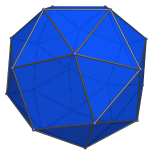The snub cube is chiral: its mirror image is distinct from itself. Its two forms are its enantiomorphs, shown below: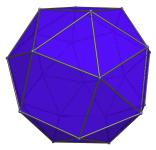The 32 triangular faces of the snub cube are of two kinds. The first kind, consisting of 8 triangles, corresponds with the vertices of the cube. These are shown below in yellow: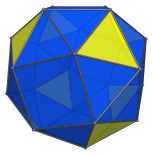The remaining 24 triangular faces come in 12 pairs, corresponding with the 12 edges of the cube. The triangles in each pair share an edge with each other. These 24 faces are shown below: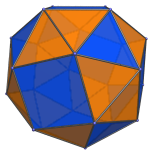To help identify the two kinds of triangular faces, we will henceforth color the triangles of the first kind slightly differently: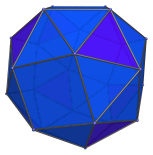## Projections

The following are projections of the snub cube from some representative viewpoints:

Projection Envelope Description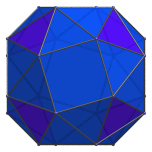Octagon

Centered on a square face.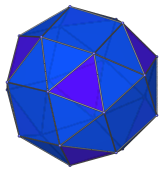Irregular dodecagon

Centered on a triangular face of the first kind. Note chiral trigonal symmetry of projection.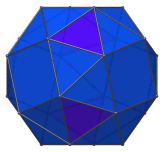Octagon

Centered on edge between pair of triangles of the second kind.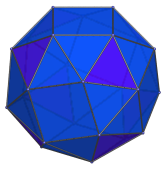Irregular nonagon

Vertex-first parallel projection.

## Coordinates

The Cartesian coordinates of the snub cube, centered on the origin and with edge length 2, are the even permutations of coordinates and even number of sign changes of:

• (√A, √B, −√C)
• (√A, √C, √B)

where A, B, and C are the roots of the following polynomials within the indicated ranges:

 A3 − 4A2 + 6A − 2 = 0, 0 < A < 1 B3 − 2B2 + 2B − 2 = 0, 1 < B < 2 C3 − 4C2 − 6C − 2 = 0, 4 < C < 6

The approximate values of √A, √B, and √C are:

• √A = 0.675507947627505
• √B = 1.242452821113171
• √C = 2.285227017851924

### Connection with the Tribonacci Constant

Scaling the coordinates by 1/√B produces coordinates of the form:

• (1, ξ, 1/ξ)

where ξ is the solution to the equation:

ξ3 + ξ2 + ξ = 1

ξ is known as the Tribonacci Constant, the cubic analogue of the Fibonacci Constant, φ=(1+√5)/2, also known as the golden ratio. The exact value of ξ is:

(∛(17+3√33) − ∛(−17+3√33) − 1)/3

Numerically, ξ is approximately 0.54368901.

A very nice explanation of how these coordinates are derived may be found on Paul Scott's snub cube page.

## Occurrences

The snub cube does not occur as cells in any uniform 4D polytopes except its own prism, the snub cube prism.

## Credits

The ξ-based Cartesian coordinates of the snub cube are described on the Wikipedia snub cube page.

Last updated 17 Jun 2019.